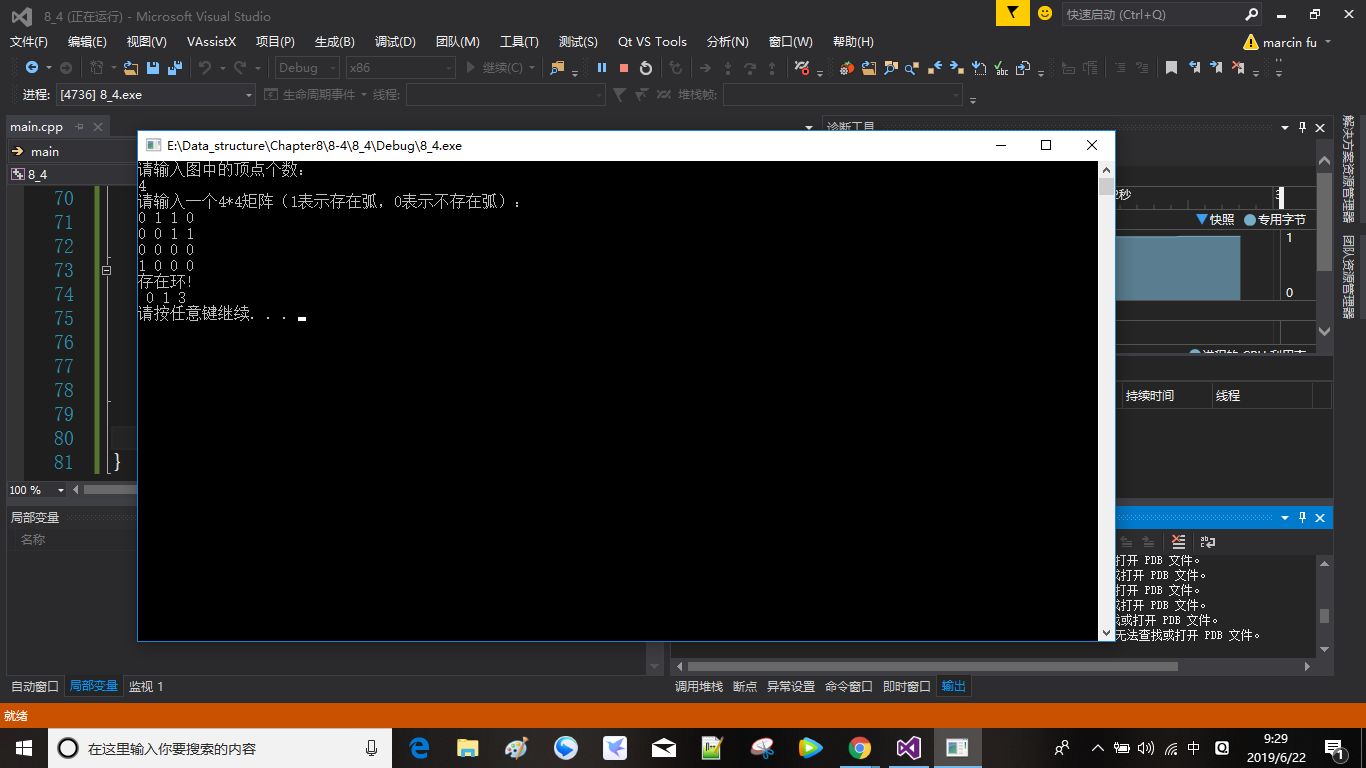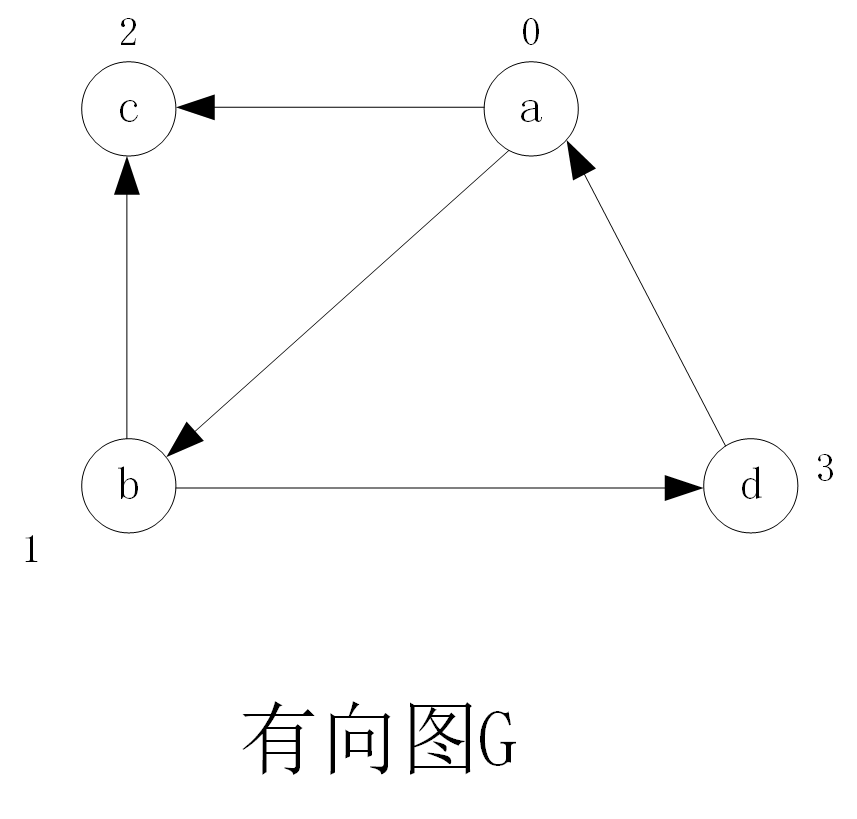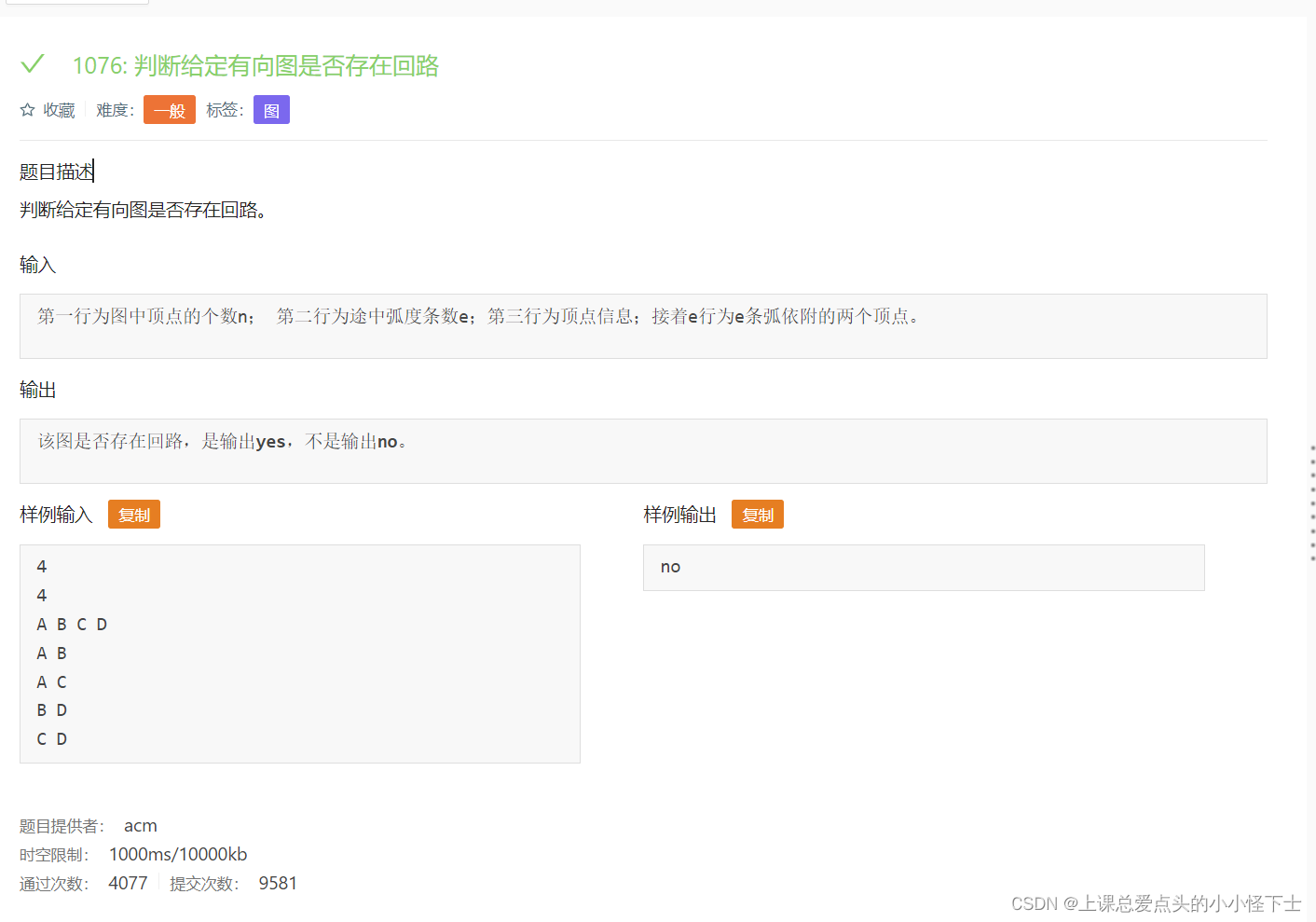• 2019-11-19 22:33:01

数据结构–判断一个图中是否有环
bool VCycle(v)
{
bool flag;

visited(v)=-1;//标记起始点

WHILE (p !=NULL)
DO
{

if( visited(vertex(p))==-1) //又回到了起点，所以，存在环

{
flag=ture;
return flag;
}

if(visited(vertex(p))==0 )//没被访问过

{
flag=VCycle(vertex(p));//判断该点是否有回路
if(flag==ture)
return flag;
}
}


visited(v)=1;//被访问过
flag=false;//该点不成环
return flag;
}
bool Cycle(G)//判断整个图是否有回路

{
Let v be the first vertex in G;//此处用英文理解吧

WHILE v is existed DO (

	IF visited(v) = 1
THEN CONTINUE


;
VCycle(v. flag) ;

	IF flag=TRUE
THEN RETURN


;
Reset the status of visited vertices

	Let v be the next vertex;)


}数据结构
更多相关内容
• 判断一个有向图中是否存在回路，并进行输出（拓扑算法）C++ 数据结构
• 假设以邻接矩阵作为的结构，编写算法，判别在给定的有向图中是否存在一个简单的有向回路，若存在，则以顶点序列的方式输出该回路（找到一条即可）（注意：中不存在顶点到自身的弧） 这是清华大学的考研试题。...

假设以邻接矩阵作为图的结构，编写算法，判别在给定的有向图中是否存在一个简单的有向回路，若存在，则以顶点序列的方式输出该回路（找到一条即可）（注意：图中不存在顶点到自身的弧）

这是清华大学的考研试题。为了判断有向图是否存在环，可以通过深度优先搜索的方法来实现。从编号0的顶点出发，若两个顶点间存在路径，则记录下源顶点标记为已访问（标记为-1）。在遍历的过程中，若有顶点与源顶点相同，则说明存在环。

code:

#include <iostream>
#include <stdlib.h>
#include <stdio.h>
#include <string.h>
#include <conio.h>
using namespace std;
const int N = 100;
int G[N][N];
int path[N], visited[N], n, cycle;
int DFS(int u, int start)
{
int i;
visited[u] = -1;
path[u] = start;
for (i = 0; i < n;i++)
{
if (G[u][i]&&i!=start)
{
if (visited[i]<0)
{
cycle = u;
return 0;

}
if (!DFS(i,u))
{
return 0;
}
}
}
visited[u] = 1;
return 1;
}

void DisPath(int u)
{
if (u<0)
{
return;
}
DisPath(path[u]);
cout << " " << u;

}

void main()
{
int i, j;
cout << "请输入图中的顶点个数：" << endl;
cin >> n;
memset(G, 0, sizeof(G));
cout << "请输入一个" << n << "*" << n << "矩阵（1表示存在弧，0表示不存在弧）：" << endl;
for (i = 0; i < n;i++)
{
for (j = 0; j < n;j++)
{
cin >> G[i][j];
}
}
cycle = -1;
for (i = 0; i < n;i++)
{
if (!visited[i]&&!DFS(i,-1))
{
break;

}
}
if (cycle<0)
{
cout << "不存在环!" << endl;
}
else
{
cout << "存在环!" << endl;
DisPath(cycle);
cout << endl;
}

system("pause");
}

结果：该算法创建4x4矩阵对应的有向图如下图所示其中，a，b，c，d分别对应的编号为0，1，2，3，有向图有5条弧：<a,b> ,<a,c>,<b,c>,<b,d>和<d,a>，因此存在环a->b->d->a

展开全文数据结构与算法
• 存储结构：邻接矩阵； 实现功能：深度遍历求回路； 博客的代码实现
• loop函数无论是强连通和非强连通均能判断是否存在回路。 在loop函数 1.visited数组用于记录被访问过的节点，和DFS算法的visited数组相同。 2.count数组只有一个元素即count，初始值为1，若能找到回路,...

判断一个有向图是否存在回路，除了采用拓扑算法以外，还可以使用深度优先搜索算法，本算法改编自“用邻接矩阵表示的深度优先搜索算法”，即DFS算法，两者共同点均为递归调用,DFS算法已在注释中标出，可进行对比学习。loop函数无论是强连通图和非强连通图均能判断出是否存在回路。

在loop函数中
1.visited数组用于记录被访问过的节点，和DFS算法中的visited数组相同。
2.count数组只有一个元素即count，初始值为1，若能找到回路,则count=1；为什么用count而不直接用int count变量？因为loop函数中要进行很多次递归调用，若用count变量，当形参改变时，实参的值并不能跟着改变，无法判断count的值是否改变。若是能找到回路，count++只执行一次，即变为1.
3.形参source为源节点，以source为源节点，loop在遍历过程中是否能回到source节点,若能回到source节点，则存在回路。
4.形参s用于记录source节点，在loop函数不断递归过程中,source的值回不断的改变，无法知道源节点是哪个，故需要一个s来记录source 节点，s在递归过程中始终不变。

void loop(GraphMatrix *graphMatrix,int *visited,int source,int s,int *count)
{
int j;
visited[source]=1//源节点被遍历到，记录为1;
for(j=0;j<graphMatrix->size;j++)
{
if(graphMatrix->graph[source][j]!=MAX&&j==s)//在每一次使用loop函数中，count++其实只执行一次
{   count++;
printf("%d",count);
}

if(graphMatrix->graph[source][j]!=MAX&&!visited[j])//若有节点还为遍历到，则继续递归
loop(graphMatrix,visited,j,s,count);
}
}

/*void DFS(GraphMatrix*graphMatrix,int* visited,int source)
{
int j;
visited[source]=1;
printf("%d",source)
for(j=0;j<graphMatrix;j++)
{
if(graphMatrix->graph[soure][j]!=MAX&&!visited[j])
DFS(graphMatrix,visited,j);
}
}*/


完整代码如下

#include <stdio.h>
#include <stdlib.h>
#define MAX 0
typedef struct GRAPHMATRIX_STRU
{
int size;
int **graph;
}GraphMatrix;

GraphMatrix* InitGraph(int num)
{
int i,j;
GraphMatrix *graphMatrix=(GraphMatrix*)malloc(sizeof(GraphMatrix));
graphMatrix->size=num;
graphMatrix->graph=(int**)malloc(sizeof(int*)*graphMatrix->size);
for(i=0;i<graphMatrix->size;i++)
graphMatrix->graph[i]=(int*)malloc(sizeof(int)*graphMatrix->size);
for(i=0;i<graphMatrix->size;i++)
{
for(j=0;j<graphMatrix->size;j++)
graphMatrix->graph[i][j]=MAX;

}
return graphMatrix;
}
{
int vex1,vex2;
scanf("%d%d",&vex1,&vex2);
while(vex1!=-1||vex2!=-1)
{
graphMatrix->graph[vex1][vex2]=1;
scanf("%d%d",&vex1,&vex2);
}

}
void loop(GraphMatrix *graphMatrix,int *visited,int source,int s,int *count)
{
int j;
visited[source]=1//源节点被遍历到，记录为1;
for(j=0;j<graphMatrix->size;j++)
{
if(graphMatrix->graph[source][j]!=MAX&&j==s)//在每一次使用loop函数中，count++其实只执行一次
{   count++;
printf("%d",count);
}

if(graphMatrix->graph[source][j]!=MAX&&!visited[j])//若有节点还为遍历到，则继续递归
loop(graphMatrix,visited,j,s,count);
}
}

/*void DFS(GraphMatrix*graphMatrix,int* visited,int source)
{
int j;
visited[source]=1;
printf("%d",source)
for(j=0;j<graphMatrix;j++)
{
if(graphMatrix->graph[soure][j]!=MAX&&!visited[j])
DFS(graphMatrix,visited,j);
}
}*/
int main()
{
int num=7;//节点个数为7个，可将num改为输入方式，节点个数动态定义
int i=0,j;
int count={0};//count=0则不存在回路，count=1则存在回路
int *visited=(int*)malloc(sizeof(int)*num);
GraphMatrix *graphMatrix;
//初始化邻接矩阵
graphMatrix=InitGraph(num);
//从0开始判断每一个节点是否能存在回路
for(i=0;i<(graphMatrix->size);i++)
{
if(count!=0)//若oount变为1，则已存在回路，不需要再进行下去了
break;
for(j=0;j<graphMatrix->size;j++)//每一次都 visited数组元素全部初始化，因为每次循环都是不同的源接待你
{
visited[j]=0;
}
loop(graphMatrix,visited,i,i,count);

}
if(count==0)
printf("0");
return 0;
}



若存在回路则输出1，否则输出0.输入输出样例如下展开全文数据结构 算法 c语言
•  若此时输出的顶点数小于有向的顶点数，则说明有向图中存在回路，否则输出的顶点的顺序即为一个拓扑序列 代码部分 #include #include #include using namespace std; int indegree, vertexs; char...

目录

题目

拓扑排序的算法步骤

代码部分

小结

## 题目## 拓扑排序的算法步骤

1. 求出所有顶点的入度，可以附设一个存放各顶点入度的数组indegree[]
2. 遍历数组indegree[],如果有顶点的入度为零，便将顶点依次入队或者入栈
3. 当栈或者队列不为空时，一直重复下面两个操作

1）进行出栈或者出队的操作，这里假设操作顶点为v

2）将与顶点v邻接的所有顶点的入度减一，如果出现入度为0的顶点，便进行入栈或者入队操作

4. 若此时输出的顶点数小于有向图的顶点数，则说明有向图中存在回路，否则输出的顶点的顺序即为一个拓扑序列

## 代码部分

#include <iostream>
#include <algorithm>
#include <queue>
using namespace std;
int indegree, vertexs;
char Data;
int main()
{
queue<int> que;
int m, n, i;
char x, y;
cin >> n >> m;
for (i = 0; i < n; i++)  cin >> Data[i]; //存入顶点数据
while (m--)
{
cin >> x >> y;
vertexs[x - 'A'][y - 'A'] = 1;
indegree[y - 'A']++; //计算每个顶点的入度
}
for (i = 0; i < n; i++)  if (!indegree[i])    que.push(i); //将入度为零的顶点编号入队
int count = 0;       //计算拓扑序列的顶点数
while (!que.empty())
{
int start = que.front();
que.pop();
count++; //相当于输出一个拓扑序列中的一个编号
for (i = 0; i < n; i++)
{
if (vertexs[start][i])
{
vertexs[start][i] = 0;
indegree[i]--;
if (!indegree[i])    que.push(i);
}
}
}
if (count == n)    cout << "no"; //存在拓扑排序,即不存在回路
else    cout << "yes"; //不存在拓扑排序，即存在回路
return 0;
}


## 小结

主要代码的解释放在了代码块里面，仅供参考，有问题可以评论区交流，有问必答！！

展开全文算法 数据结构 c++
• 思路： 一.借助AOV的拓扑排序算法来对整个有向进行排序 拓扑排序算法： ...如果恰好等于节点总数，说明此有向图中没有回路 否则说明图中回路 关键代码： int AOV::Topusort_AOV() { stack<int
• 判断给定有向图是否存在回路。 输入 第一行为图中顶点的个数n； 第二行为途中弧度条数e；第三行为顶点信息；接着e行为e条弧依附的两个顶点。 输出 该图是否存在回路，是输出yes，不是输出no。算法 深度优先 c语言 数据结构
• 判断给定有向图是否存在回路。 输入 第一行为图中顶点的个数n； 第二行为途中弧度条数e； 第二行为顶点信息;接着e行为e条弧依附的两个顶点。 输出 该图是否存在回路，是输出yes;，不是输出no。 样例输入 4 4 A B C D...数据结构 拓扑排序 队列
• 2）重复过程1），直到没有入读为0的点，如果还有没被删除的节点，则该有向一定存在回路 如果G为无向： 1）首先删除所有度数<=1的点，然后将与这些点相连的所有点的度数-1，然后将所有度数为1的点加入队列...python 数据结构 算法
• 问题描述：给一个G=，问如何判断这个图中是否存在回路？请给出至少3中方法 分析： 方法1：利用减枝的方法，如果G为有向：  1）首先删除入读为0的点，并且将对应的和该点相连的点的入读-1。（可以用一...算法
• #include #include using namespace std;...//num用来判断每个节点的度是否为偶数 for(int j=1;j;j++) { if(juzhen[i][j]==1) num++; } if(num%2!=0) {//不是偶数，就输出0返回 cout; return 0; } } cout; return 0; }dfs 数据结构
• 现给定一个，问是否存在欧拉回路？ Input 测试输入包含若干测试用例。每个测试用例的第1行给出两个正整数，分别是节点数N ( 1 < N < 1000 )和边数M；随后的M行对应M条边，每行给出一对正整数，分别是该条边...
• 提示：判别一个图是否回路，可以有以下几种方法： （1）利用深度优先遍历； （2）拓扑排序。 设计步骤： 1.建立 2.利用深度优先遍历来遍历查找 代码： #include <stdlib.h> #include <stdio.h> #...算法 深度优先
• 设快、慢两个指针：fast和slow，在程序开始时，二者都指向单链表的链表头，之后循环移动两指针，fast指针在一次循环向前移动两步（fast=fast->.../* 判断链表是否有环 */ bool isLinkedListCo
• 根据拓扑排序中是否所有点都入队（可形成拓扑序）来判断是否有回路 ​ ​ #include #include #include #include using namespace std; int main() { queueq; int cnt = 0; vectorindegree(1000,0); //数组记录每个...c++
• 拓扑排序是对测试AOV网是否存在回路的方法！ 拓扑排序的过程，由于需要查找所有以某顶点为尾的弧，即找到该顶点的所有出边，故要采用邻接表的存储方式。但拓扑排序较邻接表的存储方式有一点不同，由于要查找...
• 1.欧拉回路：定义：经过（有向或无向每条边一次且仅一次并且行遍图中每个顶点的回路（ 闭合的欧拉路径，即一个环，保证每条边都通过且仅通过一次）。 2.问题2：判断一个图是否有欧拉路径： （1）G是连通...dfs
• 判断给定有向图是否存在回路。输入第一行为图中顶点的个数n； 第二行为途中弧度条数e； 第二行为顶点信息;接着e行为e条弧依附的两个顶点。输出该图是否存在回路，是输出yes;，不是输出no。样例输入4 4A B C D A B...
• 所设计的程序能够通过编译，并能够实现从给定10个结点的G的邻接矩阵A， 判断G是否存在欧拉回路存在则输出True，否则输出False。 输入格式 首先输入G的种类，无向：输入1，有向：输入2；然后输入G的邻接...c语言
• 判断给定有向图是否存在回路 1000(ms) 10000(kb) 1465 / 3415Tags: 图判断给定有向图是否存在回路。输入第一行为图中顶点的个数n； 第二行为途中弧度条数e； 第二行为顶点信息;接着e行为e条弧依附的两个顶点。...
• 2.根据图论，如果无向弧的个数大于等于节点个数，那么必定存在环 3.把度小于2的点全部删除，并且删除与此节点相关的弧，再统计剩下节点度小于2的点，再把这些点删除，同时删除与此节点相关的弧，直到最后，形成...
• 参考王红梅、胡明、王涛编著的《数据结构（C++版）》的思想，采用并查集判断无向图是否存在回路。 //无向图判断是否有环路。 #include <iostream> using namespace std; #define N 1000 typedef struct ...并查集 C++
• 而拓扑排序的作用，就是帮我们判断一个有向图是否回路出现。 2. 拓扑排序的思想 其实拓扑排序的思想很简单： （1）在有向图中选择一个没有前驱（入度为0）的顶点输出； （2）从图中删除该顶点和所有以它为尾的弧；...数据结构 拓扑排序
• 利用深度优先遍历可以判断图G中是否存在回路。对于无向来说，若深度优先遍历过程中遇到了回边，则必定存在环；对于有向来说，这条回边可能是指向深度优先森林中另一棵生成树上的顶点的弧；但是，从有向的某个...
• 算法Cycle(G,v,has)从顶点v出发判断图G中是否存在回路，has是布尔值，初始调用时置为false，执行后若为true表示有回路，否则表示G中没有回路。 对应的算法如下： void Cycle(AGraph *G,int v,boolc语言...